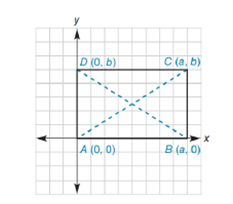Chapter 10.1, Problem 30E### Elementary Geometry for College St...

6th Edition
Daniel C. Alexander + 1 other
ISBN: 9781285195698

#### Solutions

Chapter
Section### Elementary Geometry for College St...

6th Edition
Daniel C. Alexander + 1 other
ISBN: 9781285195698
Textbook Problem
1 views

# The rectangle whose vertices are A 0, 0 ,   B a ,   0 ,   C a ,   b , and D ( 0 ,   b ) is shown. Use the Distance Formula to draw a conclusion concerning the length of the diagonals A C - and B D - .To determine

To find:

The length of the diagonals AC- and BD- of the rectangle whose vertices are A0, 0, Ba, 0, Ca, b, and D(0, b).

Explanation

Given vertices are,

A0, 0, Ba, 0, Ca, b, and D(0, b).

Sketch the points in the graph and draw the diagonals AC- and BD- as shown in below graph,

Length of the diagonals AC- and BD- can be determined using distance formula,

The distance between the two points (x1, y1) and (x2, y2) as below,

d=(x2-x1)2+(y2-y1)2

Coordinates of A(0, 0), C(a, b)

Length of AC=(a-0)2+(b-0)2

Leng

### Still sussing out bartleby?

Check out a sample textbook solution.

See a sample solution

#### The Solution to Your Study Problems

Bartleby provides explanations to thousands of textbook problems written by our experts, many with advanced degrees!

Get Started

#### If sin=13, find sin3.

Trigonometry (MindTap Course List)

#### True or False: is a geometric series.

Study Guide for Stewart's Single Variable Calculus: Early Transcendentals, 8th